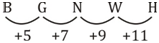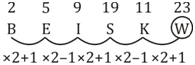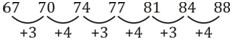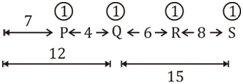# SSC Reasoning Ability Practice Questions (Day-70)

Dear Aspirants, The SSC Exams are going on at national level. Millions of candidates have eagerly prepared for the ongoing SSC exams such as CGL, CHSL, MTS, CPO, JE etc. SSC exams are usually conducted by the Staff Selection Commission to recruit the eligible candidates for various Central government departments. Mostly the selection process for all the SSC exams consists of the single or double phase of written examination based on the examination. Due to that, the written exam consisted of a certain syllabus and exam pattern. By following those regulations, the written exam has been conducted through an online mode. Based on the latest updated exam pattern and syllabus, here we have drafted the Reasoning Ability questions on a daily basis. So aspirants start your practice session with us and mould your skill in a perfect shape.

Start Quiz

1) In the following question, select the odd number from the given alternatives.

(a) 15 – 45

(b) 9 – 29

(c) 31 – 93

(d) 41 – 123

2) In the following question, select the odd number pair from the given alternatives.

(a) 8 – 72

(b) 6 – 42

(c) 12 – 156

(d) 4 – 12

Direction (3-4): Arrange the given words in the sequence in which they occur in the dictionary.

3) 1) Reputation

2) Reptile

3) Republic

4) Replicate

5) Repository

(a) 42531

(b) 43251

(c) 45312

(d) 45231

4) 1)
Habit

2) Habitat

3) Handle

4) Hammer

5) Harvest

(a) 21453

(b) 12435

(c) 21435

(d) 14253

Direction (5-6): A series is given with one term missing. Select the correct alternative from the given ones that will complete the series.

5) B, G, N, W, ?

(a) I

(b) G

(c) J

(d) H

6) B, E, I, S, K, ?

(a) W

(b) X

(c) U

(d) V

Direction (7-8): In the following question, select the missing number from the given series.

7) 67, 70, 74, 77, 81, 84, ?

(a) 87

(b) 88

(c) 86

(d) 89

8) 6, 19, 54, 167, 494, ?

(a) 1491

(b) 1553

(c) 1361

(d) 1642

9) In a row of cars, red car is 14thfrom left and 23rd from right. How many cars are there in the row?

(a) 36

(b) 37

(c) 35

(d) 34

10) In a row of people, there are 12 people before Q. There are 4 people between P and Q. There are 15 people between Q and S. If there are 8 peoplebetween S and R, then how many minimum people are there in the row?

(a) 29

(b) 32

(c) 36

(d) 37

15 × 3 = 45

9 × 3 = 27  29

31 × 3 = 93

41 × 3 = 123

8 × (8 + 1) = 72

6 × (6 + 1) = 42

12 × (12 + 1) = 156

4 × (4 + 1) = 20 12

4) Replicate

5) Repository

2) Reptile

3) Republic

1) Reputation.

1) Habit

2) Habitat

4) Hammer

3) Handle

5) Harvest6 × 3 + 1 = 19

19 × 3 – 3  = 54

54 × 3 + 5 = 167

167 × 3 – 7 = 494

494 × 3 + 9 = 1491

Total cars in the row = 14 + 23 –1 = 36